# MEASUREMENT (ESTIMATING LENGTH)

##### HELLO, WELCOME TO A NEW CLASS

Measurement is a number that describes the size or amount of something. You can measure many things like length, area, capacity, weight, temperature and time. The most common system of measurement used is the metric system

Length is the distance from one end of an object to the other end, or from one object to another. For example, the length of a letter-sized piece of paper is 11 inches. The system for measuring length include inch, foot, yard, and mile. You can use any of these measurement units to describe the length of something, but it makes more sense to use certain units for certain purposes. For example, it makes more sense to describe the length of a rug in feet rather than miles, and to describe a marathon in miles or metres rather than inches.

You may need to convert between units of measurement. For example, you might want to express your height using feet and inches (5 feet 4 inches) or using only inches (64 inches). You need to know the unit equivalents in order to make these conversions between units

Various instruments are used to measure length.  For example:

• rulers and tape measures are marked in millimetres or centimetres to measure shorter lengths accurately.
• a trundle wheel is used to measure length to the nearest metre.  Note that a counter may be attached to the trundle wheel to count metres.
• a car’s odometer often measures distance in tenths of a kilometre.

Different units of length

The standard unit of length based on the metric system is a meter (m). According to the length that needs to be measured, we can convert a meter into various units like millimeters (mm), centimeter (cm), and kilometer (km).

Centimeters and millimeters help measure smaller lengths and meters and kilometers help measure larger lengths like distance. For example, the length of the pencils can be calculated in centimeters (cm), while kilometers can measure the distance between two buildings or places.

One hundred equal divisions of a meter give a centimeter. It is written as ‘cm’. That is,

1 m = 100 cm

One thousand equal divisions of kilometer give a meter. That is,

1 km = 1,000 m

According to the length conversion charts, the different units of lengths and their equivalents are given below:

A kilometer (km), meter (m), and centimeter (cm) are the commonly used units of length.

Converting Metric Units

 Example 1 Convert the following measurements to the units indicated: a.  7 cm to mm b.  8 m to cm c.  9 km to m Solution: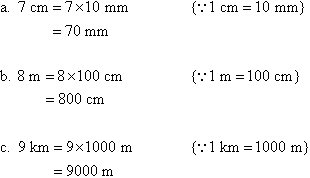Example 2 Convert the following measurements to the units indicated: a.  6 m to mm b.  4 km to cm c.  3 km to mm Solution:Example 3 Convert the following measurements to the units indicated: a.  62.8 cm to mm b.  48.7 m to cm c.  8.26 m to mm d.  4.75 km to mm Solution: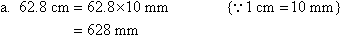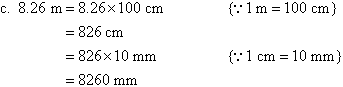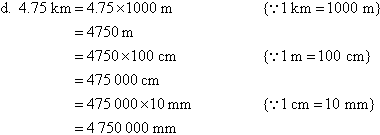Example 4 Convert the following measurements to the units indicated: a.  975 m to km b.  650 cm to m c.  8000 mm to m d.  950 000 cm to km Solution: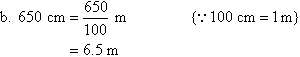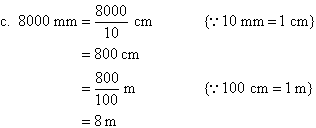Quiz

1. If we have to measure the length of a field, which type of measuring instrument will you use?
2. (i) How many metres are there in a kilometre?

(ii) How many centimetres are there in a metre?

3. Fill in the gaps:

(i) 1 km = …………………. Hectometre = ……………………… Decametre

(ii) 1 metre = ……………… Centimetre = ………………………. mm

4. Convert the following into the required measures:

(i) 10 km = ……………………… metres.

(ii) 10 metres = ………………mm

(iii) 1450 metres = ………………. km ……………………. metres.

(iv) 5680 metres = ………………. km …………………… metres

(v) 650 cms = …………………… mm.

(vi) 230 metres = ………………. cm

Your Opinion Matters! Quickly tell us how to improve your Learning Experience HERE